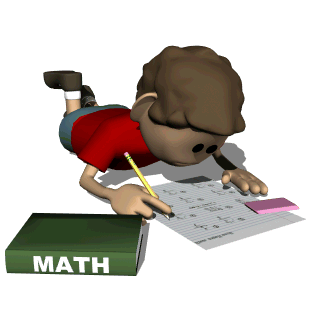• Math Objectives For First Grade

Make math a part of your daily routine. Counting can be as simple as counting pennies, cars on the road, silverware on the table, grocery items in the cart, toys picked up, and so on. Play board games to practice counting spaces. Count money found in the bottom of your purse or pocket, the amount needed to buy gum, the number of coins you can pick up with one hand. Count in the car, on a walk, everywhere! Practice telling time at bedtime, dinnertime, time to get up, time to leave for school. Tally barrettes in the drawer, toys, pennies,... Play cards games such as "war" (we call it "top-it") to practice greater than/less than and to count cards won. Most of all, make math fun!Count by 1's, 5's, 10's to 200Count by 2's, 3's, and 4's, starting at any numberCount backwards from 20 to 0Be able to identify number patterns and continue the pattern (ex-24, 34, 44,__, __,...)Be able to use tally marks to tally any numberBe able to identify numbers which are odd or even, by looking at the digit in the ones placeBe able to sequence random numbers according to their place valueAdd doubles math problems from 0 to 10 automatically (ex-1+1, 2+2, ...)Add single digit numbers by putting the highest number in ones head and adding on (ex 8+4)Add single digit numbers to a double digit number in ones head, using the add on method (ex-19+4)Add double digit numbers to double digit numbers (with no carying) on paper by adding the ones and the thens (ex 24+32)Subtract single digit numbers from single digit numbers in ones head, by using the count up method (ex-9-5). The child says the smaller number (5) and then counts up to the larger number to find the answer.Subtract single digit numbers from single digit numbers in ones head by counting backwardsSubtract a single digit number from a double digit number (no borrowing), either by counting backwards, or by subtracting the ones from the ones place (ex-28-3)Subtract double digit numbers from double digit numbers (no borrowing), by subtracting the ones column first, then the tens column (ex 47-13)Use a ruler to measure objects using standard units of measurement, such as centimeters(cm) and inches (in)Be able to tell time to the hour, half hour, and five minute intervals, on an analog clockBe able to name each coin in our currency and tell it's valueBe able to count combinations of coins and use monetary symbols to write the amounts (ex \$.56, 56 cents)Be able to identify fractional partsBe able to identify 2 and 3 dimensional shapes by name